## Calculate the Area in Excel

In Excel, you may compute the area of a parallelogram by using a conventional formula and the multiplication operator.

To get the area of a circle, use the PI function and the exponent operator (). Excel has a standard set of arithmetic operators for performing addition, subtraction, multiplication, and exponentiation when composing formulae (raising to the power of). Furthermore, Excel has operators for cell ranges, range intersections, and implicit intersections.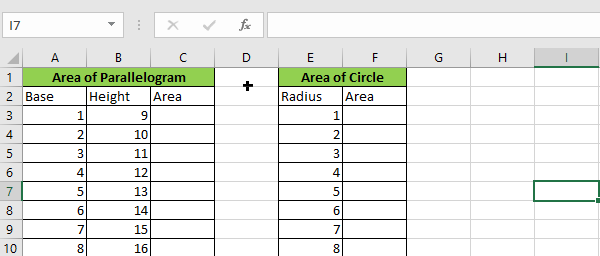## Explanation For Area of a parallelogram in Excel

A parallelogram is a four-sided quadrilateral form having two parallel pairs of sides. A parallelogram’s opposing sides are equal in length, and its opposite angles are equal in size. The area contained by a parallelogram in Euclidean geometry is determined by the formula`A=bh`, where b denotes the base and h denotes the height.

### Parallelogram Formula

As previously stated, a parallelogram is a two-dimensional structure that is studied in geometry. Each figure has its own set of attributes and settings. As a result, certain common formulae are provided for determining these characteristics.

The parallelogram formula is used to calculate both the area and perimeter of a parallelogram.

`A=base*height`

### Area of Parallelogram

The area of a figure is defined as the whole surface area occupied by it, while the area of a parallelogram is defined as the surface area covered or occupied by it. Three distinct techniques are offered for calculating the area of a parallelogram. The area of a parallelogram may be calculated using the figure’s height, side lengths, or diagonals.

The same formula may be expressed in Excel as follows:

`=b*h`

Thus, to get the area of a parallelogram with b equal to 10 and h equal to 20, we may use the following formula:

`=10*20 //it returns 200`

The objective of the example is to determine the area of eleven parallelograms by using the base value in column A and the height value in column B. The formula in C3 is as follows:

`=A3*B3`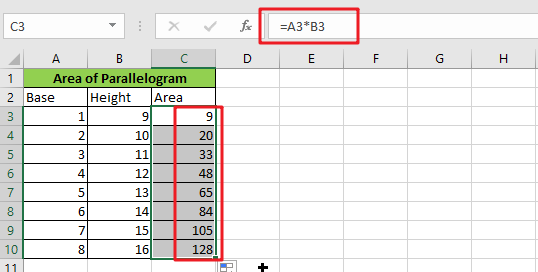Because both cells are entered as relative references, the method generates a different area for each successive row as it is copied along the table.

## Explanation For Area of a circle in Excel

The area contained by a circle of radius (r) is defined in geometry by the following formula: `πr2`

The Greek letter (“pi”) denotes the ratio of a circle’s circumference to its diameter. In Excel, the PI function returns the value 3.141592654, which is accurate to 10 digits:

`=PI() //yields a value of 3.141592654.`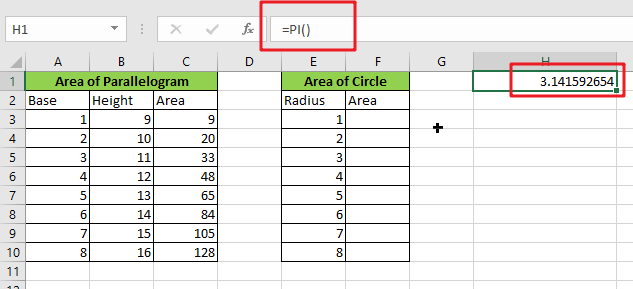In Excel, you may square an integer by using the exponentiation operator ():

`=E3^2`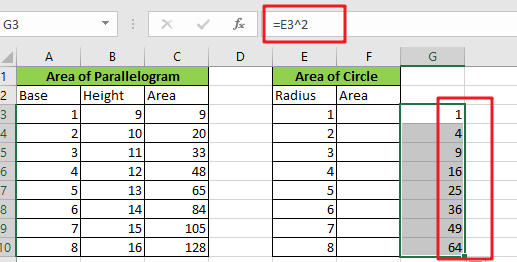Alternatively, you may use the POWER function:

`=POWER(E3,2)`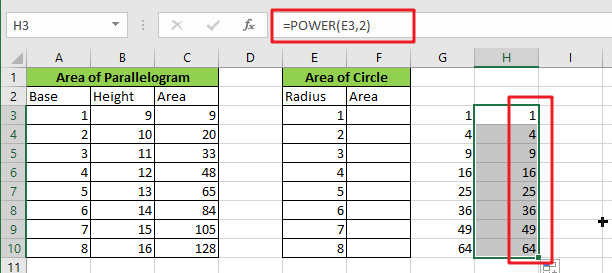By rewriting the formula `=r2 `as an Excel formula, we get the following:

`=PI()*E3^2`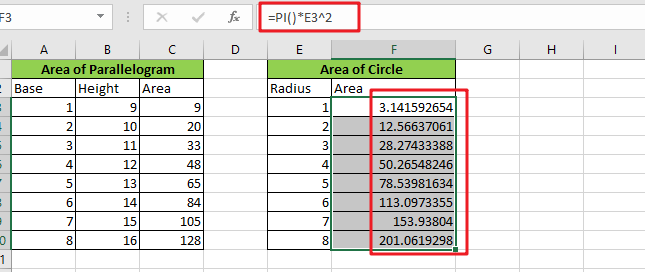Or

`=PI()*POWER(E3,2)`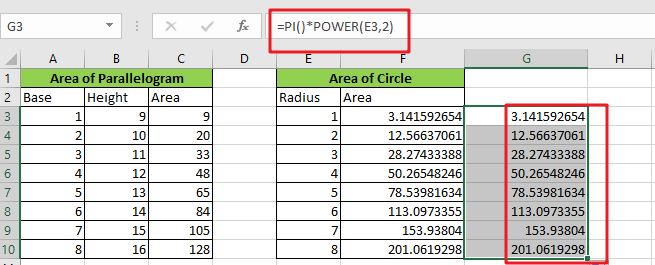Both formulae get the same outcome. Exponentiation occurs before multiplication in Excel’s sequence of operations.

### How It Operates

Pi*Radius2 is the formula for the area of a circle, where Pi is a constant roughly equal to 3.14159265. Excel includes this constant as a function with no parameter inputs called PI ().

The POWER function takes any number and multiplies it by any other number. POWER(Radius,2) returns the Radius’s square. POWER(2,2) returns a value of 4, and 3.14159265*4 equals 12.56637 in our scenario.

### Related Functions

• Excel PI function
The Excel PI function returns the value of mathematical constant PI. The returned value is 3.14159265358979 and ti will accurate to 15 digits.The syntax of the PI function is as below:=PI()…
• Excel POWER function
The Excel POWER function returns the result of a number raised to a given power.The syntax of the POWER function is as below:= POWER (number,power)….

## How to Calculate Exponential Value for a Range of Cells in Excel

This post will guide you how to calculate the exponential values for a range of cells in Excel. How to use the POWER function to do exponential calculation to a range of cells in Excel. How do I use the symbol ^ to calculate the exponential value for a range of cells in Excel. How to generate exponential series of values with known base number and exponent number in Excel.

Assuming that you have a list of data in range B1:B4 which contain numbers, and you want to calculate exponential value for those numbers in Excel. How to achieve it. You can use the symbol ^ or Power function to achieve the result.

## Calculate Exponential Value for a Range of Cells with Symbol ^

If you want the number 10 multiplied by a power of 3 in excel, it is the same as 10x10x10. You can use the symbol ^ operator to achieve the result. Just run the formula=10^3 in a blank cell. Let’s do the following steps to calculate the exponential value for the numbers in Range B1:B4 to multiply by a power of 3.

#1 select one blank cell in column C, and type the following formula in Cell C1.

=B1^3

#2 press Enter key in your keyboard, and then drag the AutoFill Handle over other cells to apply this formula.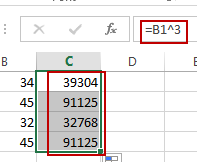## Calculate Exponential Value for a Range of Cells with Power Function

You can also use the POWER function to achieve the same result of calculate exponential value for a range of cells in Excel. Just type the following formula in blank cell C1, and press Enter key, and then drag the AutoFill Handle down to other cells to apply this formula.

=POWER(B1,3)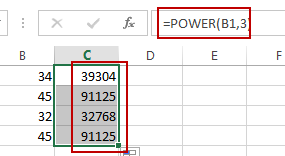Video: Calculate Exponential Value for a Range of Cells

### Related Functions

• Excel POWER function
The Excel POWER function returns the result of a number raised to a given power.The syntax of the POWER function is as below:= POWER (number,power)….

## Excel POWER Function

This post will guide you how to use Excel POWER function with syntax and examples in Microsoft excel.

### Description

The Excel POWER function returns the result of a number raised to a given power. So you can use the POWER function to calculate a given number raised to a supplied power in Excel.

The POWER function is a build-in function in Microsoft Excel and it is categorized as a Math and Trigonometry Function.

The POWER function is available in Excel 2016, Excel 2013, Excel 2010, Excel 2007, Excel 2003, Excel XP, Excel 2000, Excel 2011 for Mac.

### Syntax

The syntax of the POWER function is as below:

`= POWER (number,power)`

Where the POWER function argument are:

• number –This is a required argument. The base number that you want to raise to a power
• Power – This is a required argument. The exponent to which the base number is raised.

Note:

• The “^” operator can be used instead of POWER to indicate to what power the base number is to be raised.

### Excel POWER Function Examples

The below examples will show you how to use Excel POWER Function to calculate the result of a number raised to a power.

1# get the result of 5 squared, enter the following formula in Cell B1.

`=POWER(5,2)`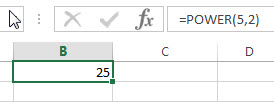2# get the result of number 45 raised to the power of 4.5, enter the following formula in Cell B2.

`=POWER(45,4.5)`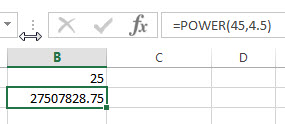3# get the result of number 23 raised to the power of 4/3, enter the following formula in Cell B3.

`=POWER(23,4/3)`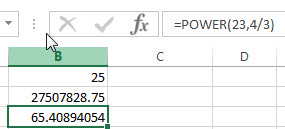## How to Calculate Compound or Average Annual Growth Rate

This post will guide you how to calculate the compound annual growth rate (CAGR) in excel. How to calculate the average annual growth rate (AAGR) using excel formula.

## What is Compound Annual Growth Rate (CAGR) and AAGR?

CAGR measures the rate of return for an investment over an investment period. It is also called a “smoothed” rate of return because it measures the growth of an investment have grown on an annual basis.

The average annual growth rate (AAGR) is the arithmetic mean of a series of annual growth rates.

## Calculate Compound Annual Growth Rate

To calculate compound annual growth rate, you need to divide the ending value of the investment by the value at the start of the period, then raise the result to the power of one divided by the number of years, and then subtract one form the subsequent result.

If you want to calculate compound annual growth rate, you can try to use the below generic CAGR formula:

`=(EV/BV)^(1/n)-1`

BV – start value of the investment

EV – End value of the investment

N – Number of years

Assuming that you have the start value in Cell B1, the ending value of the investment in cell B2, the number of periods in B3. Then we can write down the following formula to calculate CAGR:

`=(B2/B1)^(1/B3)-1`

1# enter the above formula into cell C22# select the cell you entered the formula (Cell C2), then Go to HOME Tab, click “Percent Style” command under Number group to convert the number to percentage format.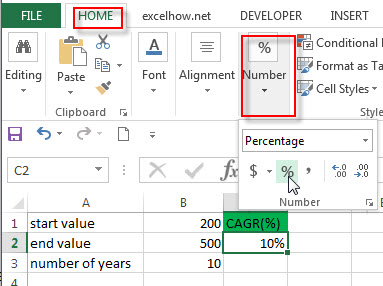You can also calculate compound annual growth rate using the POWER function to create the following generic excel formula:

`=POWER(EV/BV,1/n)-1`

For above example, you can use this generic formula to write down the following formula:

`=POWER(B2/B1,1/B3)-1`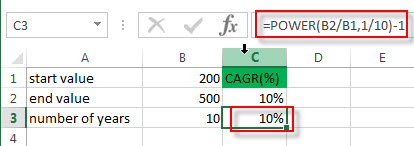There is another method for calculating CAGR in excel, you can use the RATE function to create the following generic formula:

`=RATE(n,,-BV,EV)`

N is the number of years

Bv is the beginning value of the investment

EV is the ending value of the investment

So you can enter the below formula in the Cell C2 to calculate CAGR:

`=RATE(B3,,-B1,B2)`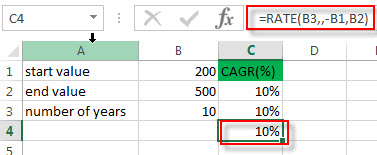## Calculate  Average Annual Growth Rate (AAGR)

You can use the below generic formula to calculate the average annual growth rate in excel.

`AAGR=(Growth rate A + Growth rate B +…)/number of years`

Assuming that you have a list of revenues for a 5-year period, you need to get the growth rate of each year firstly, such as, calculating the growth rate from 2013-2014 is (B3-B2)/B2=20%. So the generic formula of growth rate is as follows:

`Growth rate = (Ending value – Beginning Value)/ Beginning value`

And then you need to average those annual growth rates to get the AAGR value.

Let’s refer to the following steps to calculate CAAG:

1# enter the above formula of growth rate into the cell C3 to the growth rate in 2014, then press Enter key. Then drag the AutoFill Handle down to other cells.

`=(B3-B2)/B2`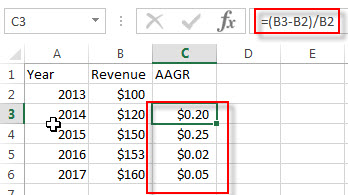2# select the range C3:C6, then go to Home Tab, click “Percent Style” command under Number group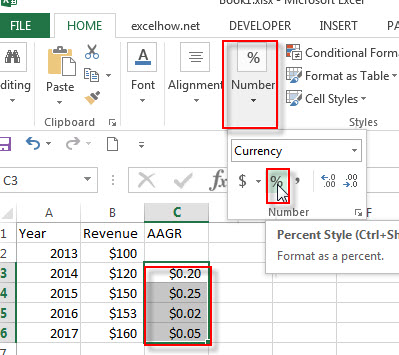3# you will see that you have the 4 annual growth rates for a 5-year period in C3:C6.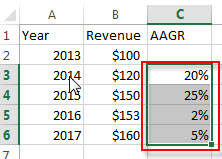4# next you need to use the AVERAGE function to average all annual growth rates to get the AAGR. So enter the below formula in Cell C7.

`=AVERAGE(C3:C6)`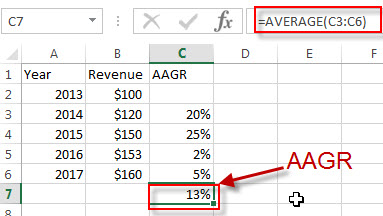### Related Functions

• Excel AVERAGE function
The Excel AVERAGE function returns the average of the numbers that you provided.The syntax of the AVERAGE function is as below:=AVERAGE (number1,[number2],…)….
• Excel RATE function
The Excel RATE function returns the interest rate per payment period of an annuity.The syntax of the RATE function is as below:=RATE(nper, pmt,pv,[fv],[type],[guess])….